# 运用 R-平方 评估策略余额曲线的品质

13 十二月 2017, 17:13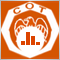0
3 511

### 评估交易系统的常用统计方法的评议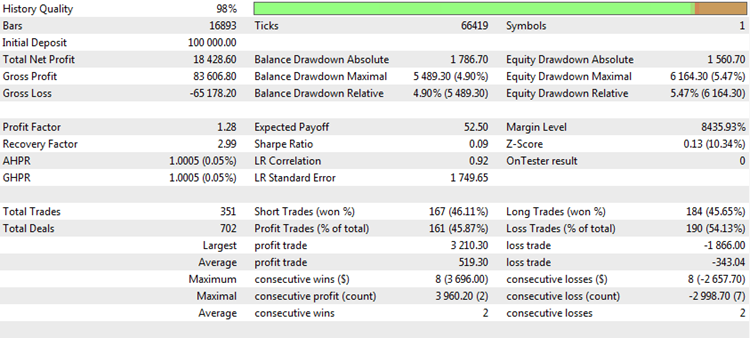Total Net Profit (净利润总计)。该衡量指标示意在测试或交易期间赚取或损失的总金额。这是最重要的交易参数之一。每位交易者的主要目标是利润最大化。有多种方法可以做到这一点, 但最终的结果总归一点, 那就是净利润。净利润并非总是取决于成交的数量, 实际上它独立于其它参数, 但反过来是不对的。因此, 相对于其它衡量指标, 它是 不变的, 所以可以独立于它们使用。不过, 这个衡量指标也有严重的缺陷。

Profit Factor (盈利因子)。这可以说是专业交易者最受欢迎的统计数据。虽说新入行者只想看总利润, 但专业人士发现知晓所投资资本的转折是必不可少的。如果认为交易亏损也是投资的一种, 那么盈利因子就展现了交易的边际性。例如, 如果只有两笔交易, 第一笔交易损失 \$1000, 第二笔交易赚取 \$2000, 则此策略的盈利因子为 \$2000/1000 = 2.0。这是一个非常良好的数字。此外, 盈利因子既不取决于测试时间跨度也未基于交易量手数。因此, 专业人士非常喜欢。但是, 它也有缺点。

Expected Payoff (预期收益)。这是一个非常重要的特征, 指明平均交易回报。如果策略有利可图, 预期收益是正数值; 亏损策略则为负数值。如果预期收益与点差或佣金成本相当, 那么这一策略在真实账户上的盈利能力就要深表怀疑。正常的话, 在策略测试器中的理想执行条件下预期收益可以为正, 余额图形可以是一条平滑的上升曲线。然而, 在实盘交易中, 由于可能出现所谓的重新报价或滑点, 平均成交回报可能比理论计算结果稍差, 这也许会对策略的结果产生严重影响, 并导致实盘亏损。

Number of Deals (成交数量)。这是一个重要的参数, 它显式或间接地影响大多数其它特性。假设一个交易系统在所有情况下的胜率为 70％。与此同时, 获胜与亏损的绝对值是相等的, 在交易策略中没有其它可能的交易结果。这样的系统似乎非常优秀, 但效率评估只基于最后两笔交易, 发生了什么呢？在 70％ 的案例中, 其中之一有利可图, 但两笔成交盈利的可能性仅为 49％。也就是说, 在一半以上的案件中, 两笔交易的总成果将为零。因此, 在一半的情况下, 统计数据将表明该策略无法赚钱。其盈利因子总是等于 1, 预期收益和利润为零, 其它参数也示意效率为零。

### 当测试交易系统时常用统计参数的行为

Total Net Profit (净利润总计)。如前所述, 净 (或总计) 利润是交易者想要得到的最终结果。利润越大越好。然而, 基于最终利润评估策略并不能保证一直成功。我们来研究一下 2015.01.15 至 2017.10.10 期间 CImpulse 2.0 策略在 EURUSD 货币对上的测试结果: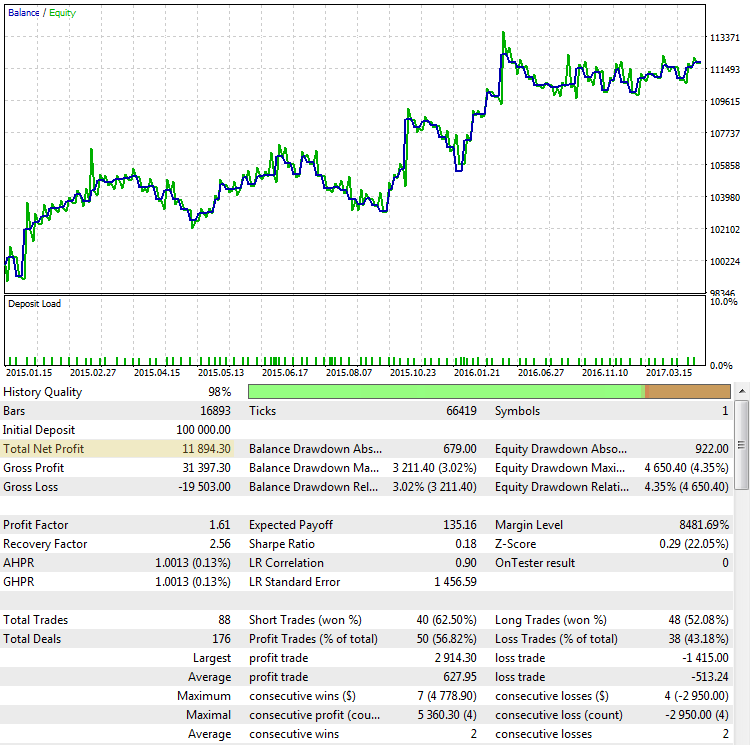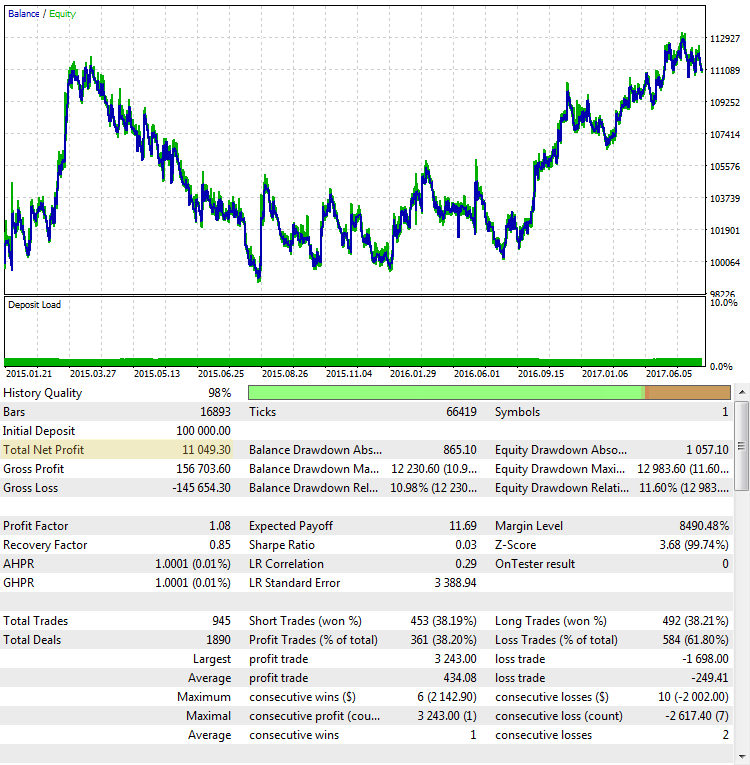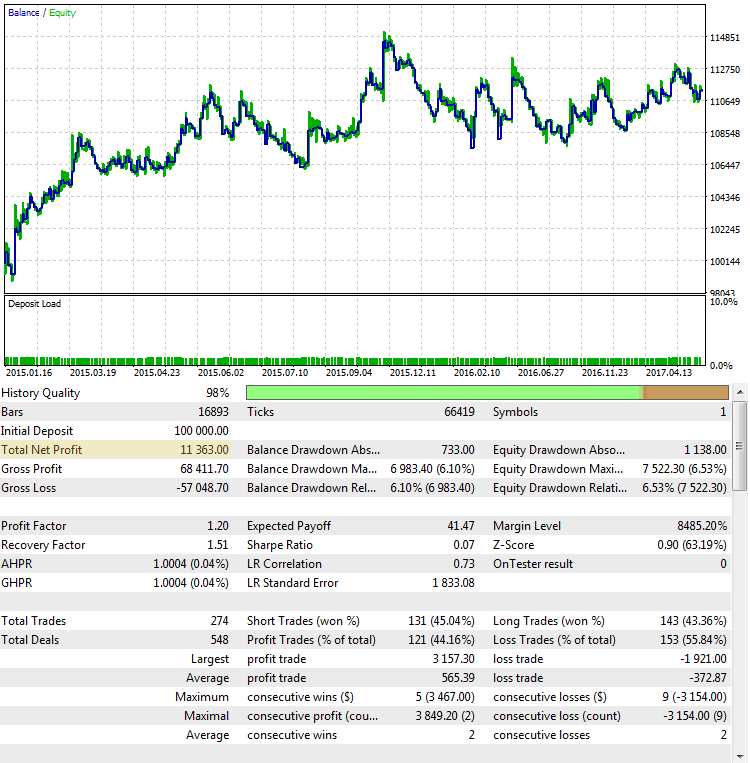Profit Factor (盈利因子)。盈利因子衡量指标对最终结果的依赖性要小得多。该数值取决于每笔成交, 并显示所有资金赢余与所有资金亏损的比率。可以看出, 在图例 2 中, 盈利因子相当高, 在图例 4 中, 它是较低的; 而在图例 3 中. 它几乎处于盈利和不盈利体系之间的边界。但尽管如此, 盈利因子并非普遍特征, 它也能被欺骗。我们来看看其它例子, 其中盈利因子指示不那么明显: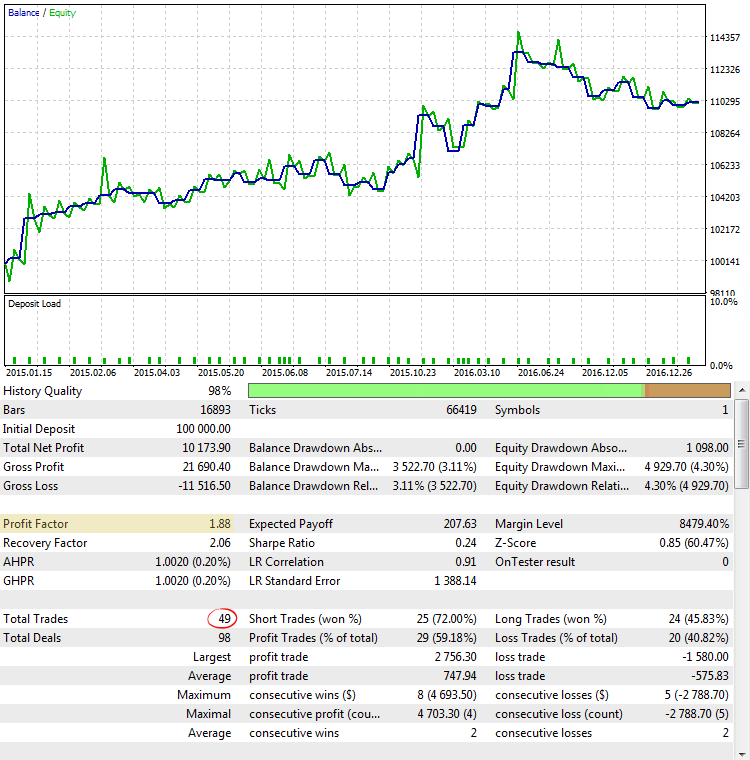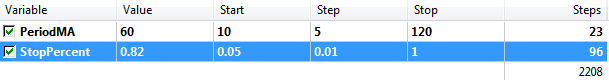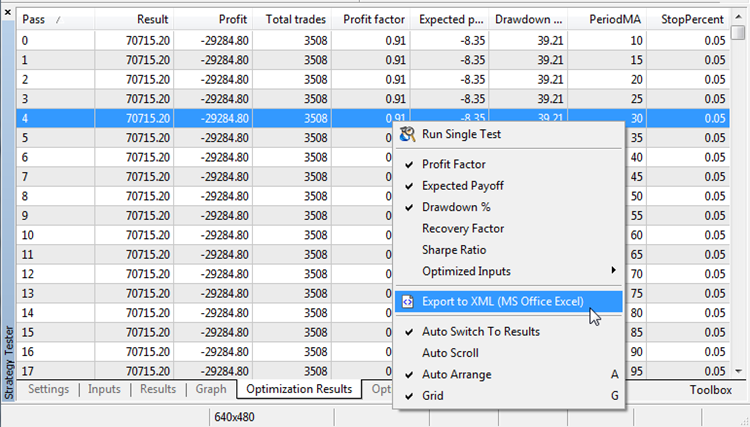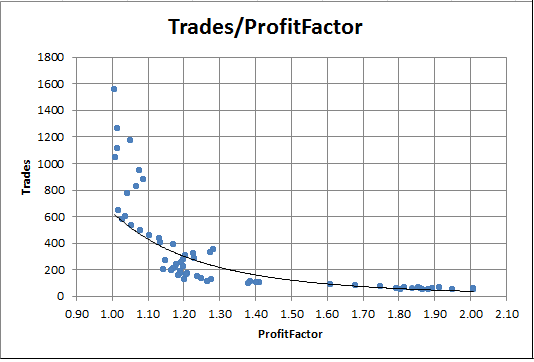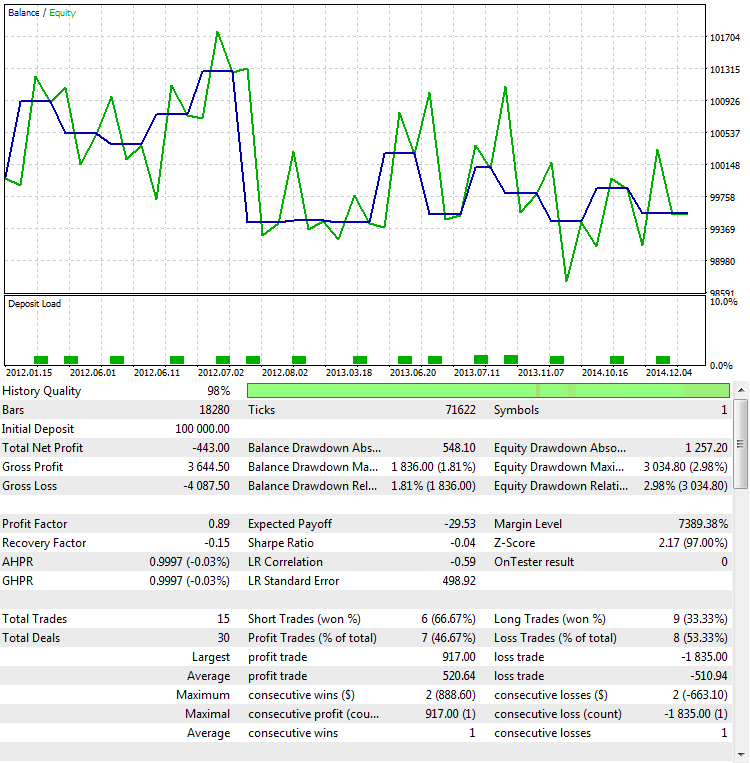Expected Payoff (预期收益)。我们不会详细讨论这个参数, 因为它的缺点与盈利因子的缺陷类似。此处是预期收益对交易数量的依赖图表: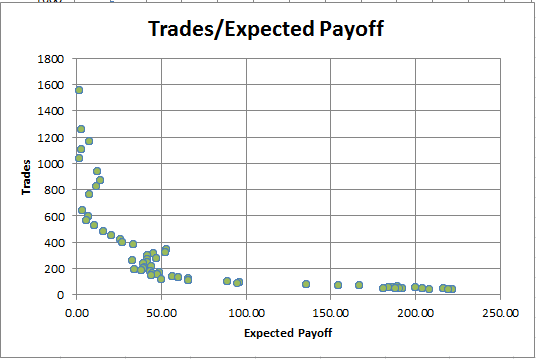### 交易系统的测试准测需求

• 独立于测试周期的持续时间。交易策略的许多参数取决于测试周期的长度。例如, 一个盈利策略的测试周期越长, 最终利润 就越大。这取决于持续时间和 恢复因子。它是计算总利润与最大回撤之比。由于利润取决于期限, 恢复因子也随着测试期限的增加而增长。相对于期限的不变性 (独立性), 必须要比较不同策略在不同测试期限的有效性;
• 独立于测试终点。例如, 如果一个策略只是通过亏损扛单才能 "保持平稳", 终点也许对最终的余额产生重大影响。如果测试在 "逾期逗留" 之时已经完成, 则浮亏 (净值) 变为余额, 并在账户上产生可观的回撤。应保护统计数据不受此类欺诈的影响, 并为交易系统运作提供客观的概述。
• 简单的释义。交易系统的所有参数都是 量化的, 即每个统计量都是由一个特定的数字表征的。这个数字必须直观。所获数值的解释越简单, 参数越易于理解。还希望参数在一定的边界范围内, 因为海量分析以及潜在的无限量数字通常很复杂。
• 少量成交所代表的结果。这可以说是衡量一个优良指标的最难要求。所有统计方法均取决于测量的次数。次数越多, 得到的统计就越稳定。当然, 少量样本完全不可能解决这个问题。然而, 可以减轻由于缺乏数据而造成的影响。为此目的, 我们开发两种类型的函数来评估 R-平方: 一种实现将基于可用成交的数量来构建这个标准。另一种是使用策略浮盈 (净值) 计算标准。

### 线性回归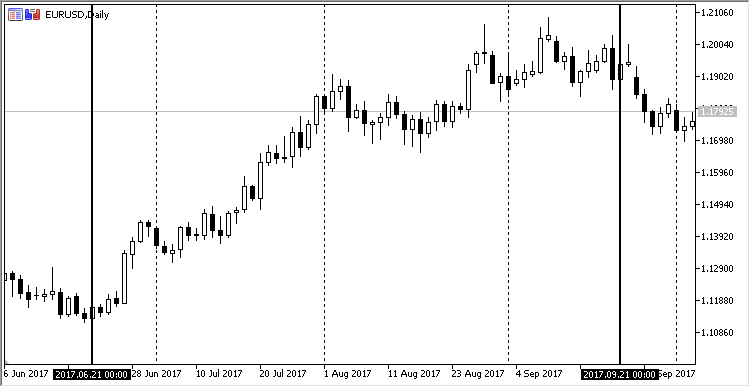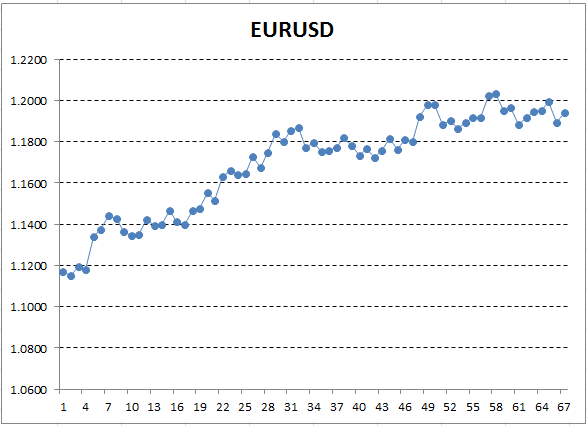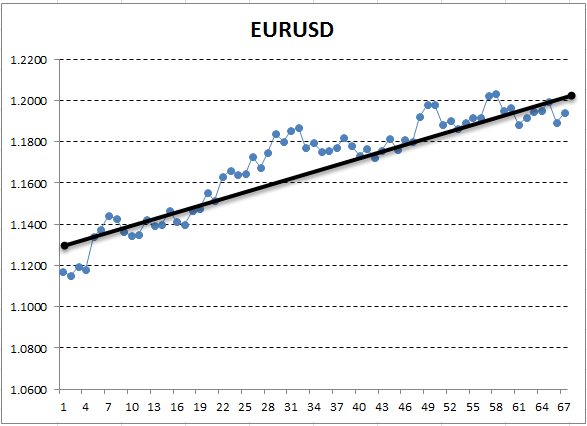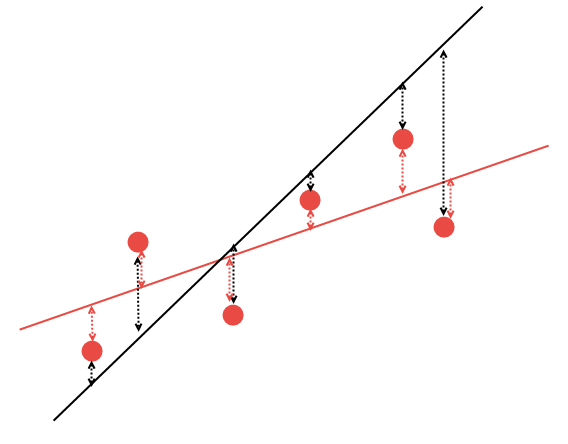### 相关性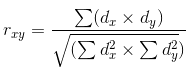MetaTrader 中的报告有一个特殊的统计指标。它被称为 LR Correlation (LR 相关性), 它表示余额曲线和线性回归之间的相关性。如果余额曲线平滑, 则直线的近似值也会很出色。在这种情况下, LR 相关性系数将接近于 1.0, 或者至少在 0.5 以上。如果余额曲线不稳定, 则上升与下降交替, 相关性系数趋于零。

LR 相关性是一个有趣的参数。但在统计学中, 通常不直接通过相关性系数来比较数据和描述的回归。其原因将在下一节讨论。

### 判定系数 R^2

R 语言的源代码详述了正在完成的事情:

```x <- rnorm(1000)            # 生成 1000 随机数, 根据正常规律分布
# 它们的方差等于一, 期望值为零
rwalk <- cumsum(x)          # 累加这些数字, 得到一个经典的随机漫游图形
plot(rwalk, type="l", col="darkgreen")       # 以线性图的形式显示数据
rws <- lm(rwalk~c(1:1000))  # 绘制线性模型 y=a*x+b, 其中 x 是测量的数量, y 是生成的漫游矢量的值
title("随机漫游的线性回归")
abline(rws)                 # 在图表上显示所得到的线性回归

```

rnorm 函数每次都返回不同的数据, 所以如果您想重复这个实验, 图形将会有不同的模样。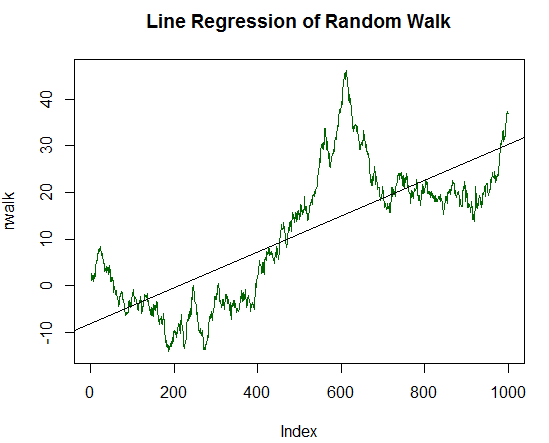```summary(rws)
Call:
lm(formula = rwalk ~ c(1:1000))

Residuals:
Min      1Q  Median      3Q     Max
-16.082  -6.888  -1.593   4.174  30.787

Coefficients:
Estimate Std. Error t value Pr(>|t|)
(Intercept) -8.187185   0.585102  -13.99   <2e-16 ***
c(1:1000)    0.038404   0.001013   37.92   <2e-16 ***
---
Signif. codes:  0 ‘***’ 0.001 ‘**’ 0.01 ‘*’ 0.05 ‘.’ 0.1 ‘ ’ 1

Residual standard error: 9.244 on 998 degrees of freedom
Multiple R-squared:  0.5903,    Adjusted R-squared:  0.5899
F-statistic:  1438 on 1 and 998 DF,  p-value: < 2.2e-16

```

```x_trend1 <- x+(sd(x)/20.0)      # 找到 x 的标准方差, 将其除以 20.0, 并将得到的数值加到每个 x 值中
# x 的每个这样的修改值将被存储在新的数值向量 x_trend1 当中
rwalk_t1 <- cumsum(x_trend1)    # 累加这些数字, 得到一个偏移的随机漫游图形
plot(rwalk_t1, type="l", col="darkgreen")        # 将数据显示为线性图
title("随机漫游 #2 的线性回归")
rws_t1 <- lm(rwalk_t1~c(1:1000))# 绘制线性模型 y=a*x+b, 其中 x 是测量的数量, y 是生成的漫游矢量的值
abline(rws_t1)                  # 在图表上显示所得到的线性回归

```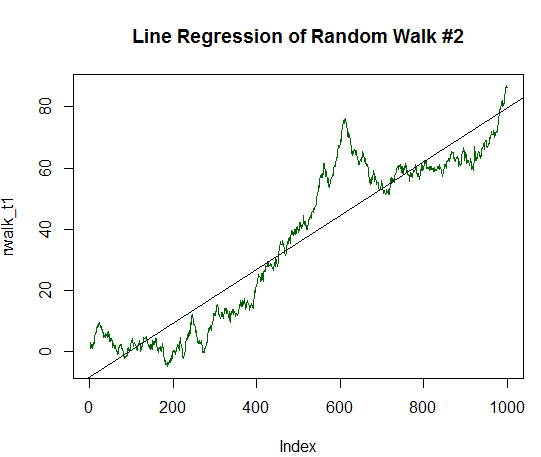```summary(rws_t1)

Call:
lm(formula = rwalk_t1 ~ c(1:1000))

Residuals:
Min      1Q  Median      3Q     Max
-16.082  -6.888  -1.593   4.174  30.787

Coefficients:
Estimate Std. Error t value Pr(>|t|)
(Intercept) -8.187185   0.585102  -13.99   <2e-16 ***
c(1:1000)    0.087854   0.001013   86.75   <2e-16 ***
---
Signif. codes:  0 ‘***’ 0.001 ‘**’ 0.01 ‘*’ 0.05 ‘.’ 0.1 ‘ ’ 1

Residual standard error: 9.244 on 998 degrees of freedom
Multiple R-squared:  0.8829,    Adjusted R-squared:  0.8828
F-statistic:  7526 on 1 and 998 DF,  p-value: < 2.2e-16

```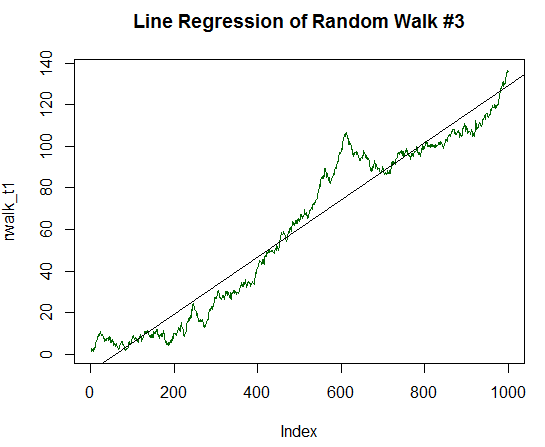```Call:
lm(formula = rwalk_t1 ~ c(1:1000))

Residuals:
Min      1Q  Median      3Q     Max
-16.082  -6.888  -1.593   4.174  30.787
4
Coefficients:
Estimate Std. Error t value Pr(>|t|)
(Intercept) -8.187185   0.585102  -13.99   <2e-16 ***
c(1:1000)    0.137303   0.001013  135.59   <2e-16 ***
---
Signif. codes:  0 ‘***’ 0.001 ‘**’ 0.01 ‘*’ 0.05 ‘.’ 0.1 ‘ ’ 1

Residual standard error: 9.244 on 998 degrees of freedom
Multiple R-squared:  0.9485,    Adjusted R-squared:  0.9485
F-statistic: 1.838e+04 on 1 and 998 DF,  p-value: < 2.2e-16

```

R-平方变得更高, 数额达至 0.9485。这幅图形与所期望的可盈利交易策略的余额动态非常相像。我们再次多走一些里程。将预期值增加到标准方差的 1/5: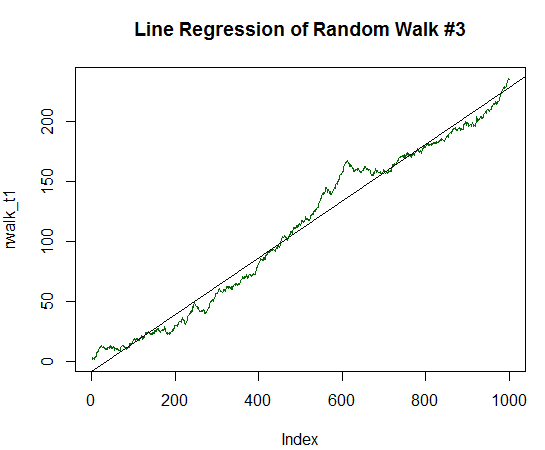```Call:
lm(formula = rwalk_t1 ~ c(1:1000))

Residuals:
Min      1Q  Median      3Q     Max
-16.082  -6.888  -1.593   4.174  30.787

Coefficients:
Estimate Std. Error t value Pr(>|t|)
(Intercept) -8.187185   0.585102  -13.99   <2e-16 ***
c(1:1000)    0.236202   0.001013  233.25   <2e-16 ***
---
Signif. codes:  0 ‘***’ 0.001 ‘**’ 0.01 ‘*’ 0.05 ‘.’ 0.1 ‘ ’ 1

Residual standard error: 9.244 on 998 degrees of freedom
Multiple R-squared:  0.982,     Adjusted R-squared:  0.982
F-statistic: 5.44e+04 on 1 and 998 DF,  p-value: < 2.2e-16

```

### 反正弦定理及其对线性回归评估的贡献

```sample_r2 <- function(samples = 100, nois = 1000)
{
lags <- c(1:nois)
r2 <- 0  # R^2 rating
lr <- 0  # Line Correlation rating
for(i in 1:samples)
{
white_nois <- rnorm(nois)
walk <- cumsum(white_nois)
model <-  lm(walk~lags)
summary_model <- summary(model)
r2[i] <- summary_model\$r.squared*sign(walk[nois])
lr[i] <- (summary_model\$r.squared^0.5)*sign(walk[nois])
}
m <- cbind(r2, lr)
}

```

```ms <- sample_r2(10000, nois=1000)
hist(ms[,2], breaks = 30, col="darkgreen", density = 30, main = "Distribution of LR-Correlation")

```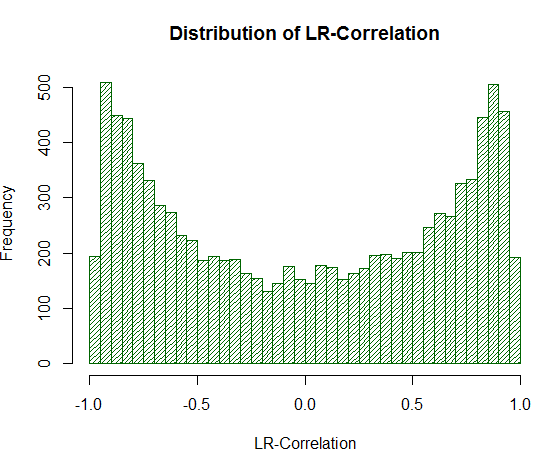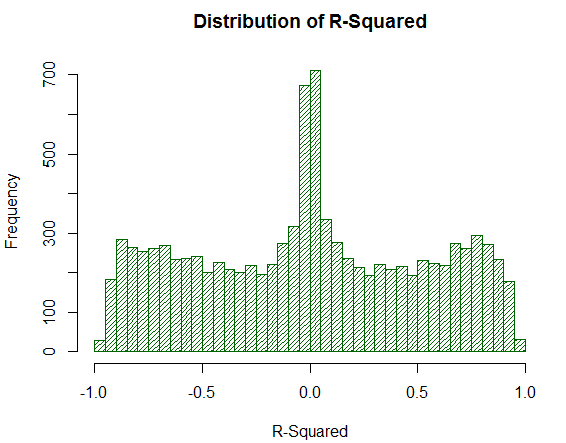### 收集策略净值

• 统计数据收集的频率
• 事件的检测, 接收那个需要检查的净值。

```//+------------------------------------------------------------------+
//|                                                UsingTrailing.mqh |
//|                                   版权所有 2017, Vasiliy Sokolov. |
//|                                             https://www.mql5.com |
//+------------------------------------------------------------------+
#property copyright "版权所有 2017, Vasiliy Sokolov."
#include "TimeSeries.mqh"
#include "Strategy.mqh"
//+------------------------------------------------------------------+
//| 集成到策略的投资组合中作为智能系统                                 |
//| 并记录投资组合的净值                                              |
//+------------------------------------------------------------------+
class CEquityListener : public CStrategy
{
private:
//-- 记录频率
CTimeSeries       m_equity_list;
double            m_prev_equity;
public:
CEquityListener(void);
virtual void      OnEvent(const MarketEvent& event);
void              GetEquityArray(double &array[]);
};
//+------------------------------------------------------------------+
//| 设置默认频率                                                      |
//+------------------------------------------------------------------+
CEquityListener::CEquityListener(void) : m_prev_equity(EMPTY_VALUE)
{
}
//+------------------------------------------------------------------+
//| 收集投资组合净值, 监视所有可能的                                   |
//| 事件                                                             |
//+------------------------------------------------------------------+
void CEquityListener::OnEvent(const MarketEvent &event)
{
if(!IsTrackEvents(event))
return;
double equity = AccountInfoDouble(ACCOUNT_EQUITY);
if(equity != m_prev_equity)
{
m_prev_equity = equity;
}
}
//+------------------------------------------------------------------+
//| 以双精度类型数组形式返回净值                                       |
//+------------------------------------------------------------------+
void CEquityListener::GetEquityArray(double &array[])
{
m_equity_list.ToDoubleArray(0, array);
}

```

R^2 对长时间没有交易的情况作出反应。在这种情况下, 不变的净值将被记录下来。净值图形成所谓的 "阶梯"。为了防止这种情况, 该方法会比较当前数值与前值。如果数值匹配, 则跳过该记录。因此, 只有净值的变化才会进入清单。

```//+------------------------------------------------------------------+
//| 确定优化后计算的自定义标准的                                       |
//| 类型。                                                           |
//+------------------------------------------------------------------+
enum ENUM_CUSTOM_TYPE
{
CUSTOM_NONE,                     // 自定义标准未计算
CUSTOM_R2_BALANCE,               // R^2 基于策略余额
CUSTOM_R2_EQUITY,                // R^2 基于策略净值
};

```

```//+------------------------------------------------------------------+
//| 设置 R^2 作为优化标准。系数为                                     |
//| 计算出的交易结果。                                                |
//+------------------------------------------------------------------+
void CStrategyList::SetCustomOptimizeR2Balance(ENUM_CORR_TYPE corr_type)
{
m_custom_type = CUSTOM_R2_BALANCE;
m_corr_type = corr_type;
}
//+------------------------------------------------------------------+
//| 设置 R^2 作为优化标准。系数为                                     |
//| 基于记录的净值计算。                                              |
//+------------------------------------------------------------------+
void CStrategyList::SetCustomOptimizeR2Equity(ENUM_CORR_TYPE corr_type)
{
m_custom_type = CUSTOM_R2_EQUITY;
m_corr_type = corr_type;
}

```

```//+------------------------------------------------------------------+
//| 相关性类型                                                        |
//+------------------------------------------------------------------+
enum ENUM_CORR_TYPE
{
CORR_PEARSON,     // 皮尔逊相关性
CORR_SPEARMAN     // 斯皮尔曼的排位顺序相关性
};

```

```//+------------------------------------------------------------------+
//| 返回基于策略余额的 R^2 估值                                       |
//+------------------------------------------------------------------+
double CustomR2Balance(ENUM_CORR_TYPE corr_type = CORR_PEARSON);
//+------------------------------------------------------------------+
//| 返回基于策略净值的 R^2 估值                                        |
//| 传递 "权益" 数组作为净值                                           |
//+------------------------------------------------------------------+
double CustomR2Equity(double& equity[], ENUM_CORR_TYPE corr_type = CORR_PEARSON);

```

```double OnTester()
{
return CustomR2Balance();
}

```

```//+------------------------------------------------------------------+
//| 增加对净值的监控                                                  |
//+------------------------------------------------------------------+
double CStrategyList::OnTester(void)
{
switch(m_custom_type)
{
case CUSTOM_NONE:
return 0.0;
case CUSTOM_R2_BALANCE:
return CustomR2Balance(m_corr_type);
case CUSTOM_R2_EQUITY:
{
double equity[];
m_equity_exp.GetEquityArray(equity);
return CustomR2Equity(equity, m_corr_type);
}
}
return 0.0;
}

```

### 利用 AlgLib 计算判定系数 R^2

• 获取净值的数值并将其转换为矩阵 M[x, y], 其中 x 是测量的次数, y 是净值。
• 对于获得的矩阵, 计算线性回归方程的 a 和 b 系数。
• 为每个 X 生成线性回归值并将其放入数组中。
• 用两个相关性公式之一找出线性回归与净值的相关性系数。
• 计算 R^2 和其符号。
• 将 R^2 的常规化数值返回给调用函数。

```//+------------------------------------------------------------------+
//| 返回基于策略净值的 R^2 估值                                        |
//| 传递 "权益" 数组作为净值                                           |
//+------------------------------------------------------------------+
double CustomR2Equity(double& equity[], ENUM_CORR_TYPE corr_type = CORR_PEARSON)
{
int total = ArraySize(equity);
if(total == 0)
return 0.0;
//-- 填充矩阵: Y - 净值, X - 数值的序号
CMatrixDouble xy(total, 2);
for(int i = 0; i < total; i++)
{
xy[i].Set(0, i);
xy[i].Set(1, equity[i]);
}
//-- 找出线性模型 y=a*x+b 的系数 a 和 b;
int retcode = 0;
double a, b;
CLinReg::LRLine(xy, total, retcode, a, b);
//-- 为每个 X 生成线性回归值;
double estimate[];
ArrayResize(estimate, total);
for(int x = 0; x < total; x++)
estimate[x] = x*a+b;
//-- 找出数值与其线性回归的相关性系数
double corr = 0.0;
if(corr_type == CORR_PEARSON)
corr = CAlglib::PearsonCorr2(equity, estimate);
else
corr = CAlglib::SpearmanCorr2(equity, estimate);
//-- 计算 R^2 和其符号
double r2 = MathPow(corr, 2.0);
int sign = 1;
if(equity > equity[total-1])
sign = -1;
r2 *= sign;
//-- 将 R^2 估值常规化到百分位 (小数点后 2 位) 之内, 并返回
return NormalizeDouble(r2,2);
}

```

```//+------------------------------------------------------------------+
//| 返回基于策略余额的 R^2 估值                                       |
//+------------------------------------------------------------------+
double CustomR2Balance(ENUM_CORR_TYPE corr_type = CORR_PEARSON)
{
HistorySelect(0, TimeCurrent());
double deals_equity[];
double sum_profit = 0.0;
int current = 0;
int total = HistoryDealsTotal();
for(int i = 0; i < total; i++)
{
ulong ticket = HistoryDealGetTicket(i);
double profit = HistoryDealGetDouble(ticket, DEAL_PROFIT);
if(profit == 0.0)
continue;
if(ArraySize(deals_equity) <= current)
ArrayResize(deals_equity, current+16);
sum_profit += profit;
deals_equity[current] = sum_profit;
current++;
}
ArrayResize(deals_equity, current);
return CustomR2Equity(deals_equity, corr_type);
}

```

```//+------------------------------------------------------------------+
//| 测试器事件                                                        |
//+------------------------------------------------------------------+
double OnTester()
{
Manager.SetCustomOptimizeR2Balance(CORR_PEARSON);
return Manager.OnTester();
}

```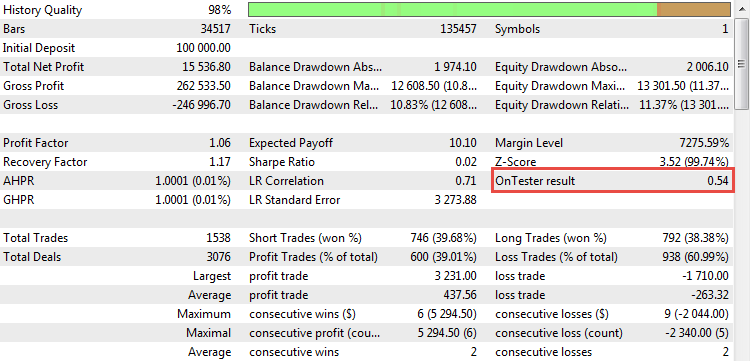### 在实际当中使用 R-平方参数

• 品名: EURUSD
• 时间帧: H1
• 期限: 2015.01.03 - 2017.10.10

EA 参数的范围列在表中:

PeriodMA 15 5 200 38
StopPercent 0.1 0.05 1.0 19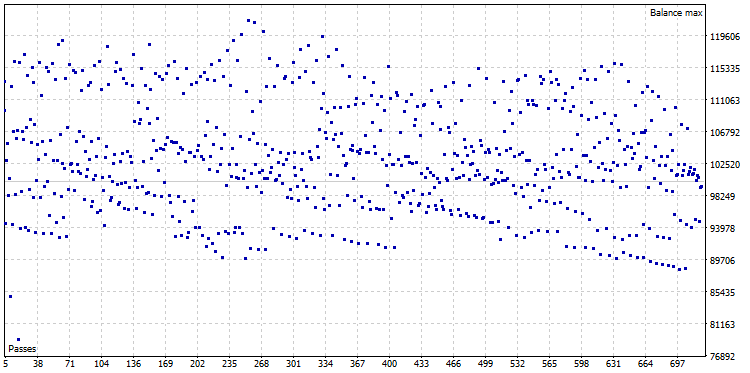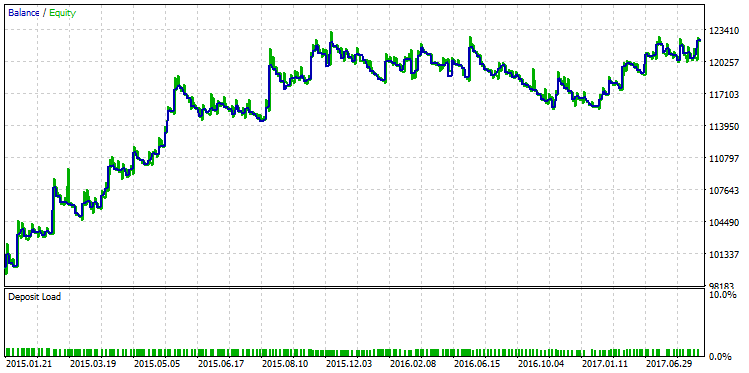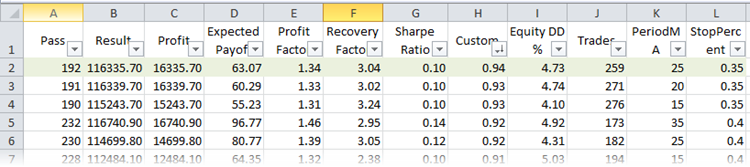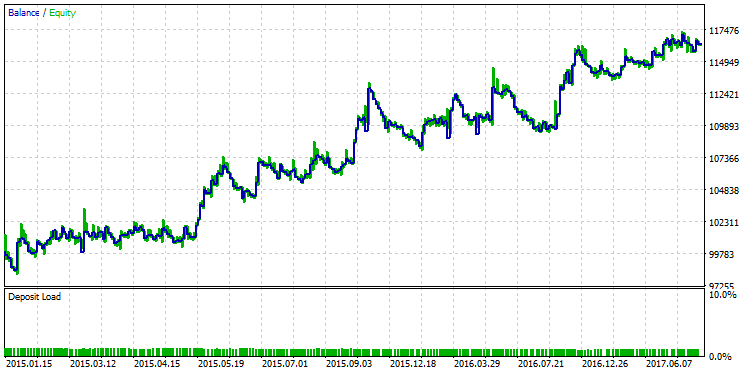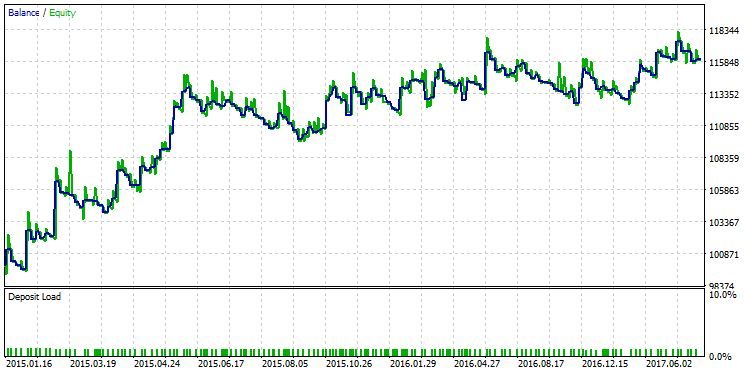### 结束语

• 用于评估交易系统的标准统计参数具有已知的缺点, 必须予以考虑。
• 在 MetaTrader 5 的标准衡量指标中, 只有 LR 相关性被设计用来评估策略余额曲线的平滑度。然而, 其数值往往被高估。
• R-平方是计算策略余额曲线和浮盈曲线平滑度的少数指标之一。同时, R-平方不存在 LR 相关性的缺点。
• 利用 AlgLib 数学库计算 R-平方。算法本身有很多修改, 在相应的例子中有详细的描述。
• 自定义优化标准可以内置到智能交易系统当中, 以便所有智能系统都可以自动计算该衡量指标, 而无需它们参与。如何执行此操作的说明已在 R-平方集成到 CStrategy 交易引擎的示例中提供了。
• 可以使用类似的集成方法计算自定义统计数据所需的附加数据。对于 R-平方, 这些数据是策略 (净值) 的浮盈数据。浮盈的动态记录由 CStrategy 交易引擎执行。
• 判定系数允许选择含有余额/净值增长的策略。在这种情况下, 选择基于其它参数的过程可能会错过这样的变体。
• 与任何其它统计衡量指标一样, R-平方也有其缺点, 在使用此值时必须考虑到这一点。

UnExpert.zip (114.84 KB)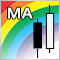交易中不同类型移动平均线的比较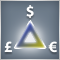三角套利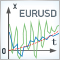利用卡尔曼 (Kalman) 滤波器预测价格方向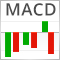将入场信息解析到指标